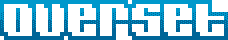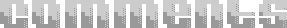CURRENT PROJECTS
CATEGORIES AND POSTSDEVELOPMENT LOG FOR JIM PALMER
Posted 07/11/2007 in javascript

Use jQuery's jQuery.extend( TRUE, target, object1, [objectN] ) "DEEP EXTEND" instead (only works in version >= 1.2.6)
```var tt = {one:[{two:1}]};
var ttt = \$.extend(true, {}, tt);
alert(ttt.one.two == tt.one.two);	// output true
ttt.one.two = 2;
alert(ttt.one.two == tt.one.two);	// output false```

update 11/5/2007
• Fixed problem where arrays were turning into objects
• Fixed problem with booleans using recursion when the shouldn't have been
So a "problem" in Javascript is that there is no direct access to passing by value for certain objects like there is in C. There's no easy way to setup a pointer or even write a custom "=" operator overload function. Although the default pass by reference in Javascript for objects, arrays, functions, etc. (non "simple" data types) passing by value is needed. Hence this nifty function that basically handles a recursive copy of objects.

I must disclaim that it does not handle 'function' typeof()'s - but it works for simple objects within objects within objects, and arrays of objects of arrays of objects. You get the idea. This could easily be modified to copy functions but I left it out so that it wouldn't copy over the prototyped json functions that I use all over the application via http://www.json.org/js.html.

Here's the code:
```function deepObjCopy (dupeObj) {
var retObj = new Object();
if (typeof(dupeObj) == 'object') {
if (typeof(dupeObj.length) != 'undefined')
var retObj = new Array();
for (var objInd in dupeObj) {
if (typeof(dupeObj[objInd]) == 'object') {
retObj[objInd] = deepObjCopy(dupeObj[objInd]);
} else if (typeof(dupeObj[objInd]) == 'string') {
retObj[objInd] = dupeObj[objInd];
} else if (typeof(dupeObj[objInd]) == 'number') {
retObj[objInd] = dupeObj[objInd];
} else if (typeof(dupeObj[objInd]) == 'boolean') {
((dupeObj[objInd] == true) ? retObj[objInd] = true : retObj[objInd] = false);
}
}
}
return retObj;
}```